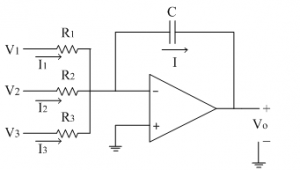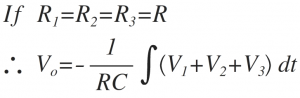Home > analog integrated circuits > op amp integrator > summing integrator

# Summing Integrator

The following figure shows the configuration of op-amp as a summing integrator.From the circuit diagram, the currents I1, I2 and I3 are added at the inverting terminal of op-amp. Since input current to the op-amp is zero, the whole current flows through the capacitor C connected in feedback path as 'I'.
The non-inverting terminal of op-amp is at ground potential. Due to virtual ground concept, the inverting terminal also appears to be at ground i. e. 0V
Thus applying KCL at inverting terminal of op-amp.
I=I1+I2+I3
Substituting the values of currents,Integrating on both the sides, we get,Thus the output equation gives the integration of addition of three input signals V1, V2 and V3. Thus acts as a summing integrator.